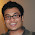### Digit Permutation Puzzle

Source: Taken from Thomer's Puzzle Website (http://thomer.com/riddles/)

Problem:
You have a number that consists of 6 different digits. This number multiplied by 2, 3, 4, 5, and 6 yields, in all cases, a new 6-digit number, which, in all cases, is a permutation of the original 6 different digits. What's the number?

1.This can be solved by simple induction if there is a solution.

let x=d5d4d3d2d1d0, d5 can only be 1, since d5*6<10.
Since d5*6+floor(d4*6/10)<10, d4<=4. Let's try to plug in d4=0,1,2,3,4.

First, if d4=0, we have contradiction, since the MSD of x, 2x,3x,... 6x will be 1,2,3,4,5,6, so there is no 0 on the list.

If d4=1, we have the MSD of x,2x,...,6x to be 1 to 6, which lead to contradiction, since x has two 1's as d5 and d4.

If d4=2,3 and 4, we have the similar contradiction.

So either there was something not clear in the puzzle, or I understood it wrong.

2.If N = d5 d4 d3 d2 d1 d0, as pointed out already d5 = 1. Therefore the number N is in the range (102345, 16598). Also, the only way in which one can get 1 in the new permutation of N when multiplied by 2 is to have 5 in the number N. I was not able to proceed beyond these two arguments. However based on raw simulation, the answer is 142857. And surprisingly (at least for me), the outputs when multiplied by 2,3,4,5,6 are cyclic version of the original number N.

3.I stated it wrong. The answer should be 142857.

4.142857 !

or 999999/7

5.Ok, quite a lengthy analysis.

First digit obviously must be 1.

Fix the LST to be any numeral and see what different LST result when multiplied by 1,2,3,4,5,6.

1: LST cannot be 1

2: 4,6,8,0,2, carry of 8*2 and 6*2 to next significant digit results in odd numerals, one covers '1', other one leads to contradiction

3: 6,9,2,5,8, no 1 hence cannot be

4: 8,2,6,0,4 : same as for 2

5: 0,5,0,5,0
But my analysis is case by case and very lengthy. so i skip

6: 2,8,4,0,6, same as for 2

7: 4,1,8,5,2 no immediate contradictions, so consider this later

8: 6,4,2,0,8, same as for 2

9: 8,7,6,5,4, no 1, hence wrong

0: 0,0,0,0,0, cannot happen, again case by case and big, hence skipped.

now remaining, 7,4,1,8,5,2

i.e. 1 [2 4 5 8] 7
1 must be succeeded by 2 or 4
similarly for second digit
hence 12___7 or 14___7
12___7 : then 4 will be followed by 5/8 resulting in 9 on product with 2. Hence cannot be
14___7 : 4 should be followed by 2
142__7 : only two cases
142587, 142857
142597*3=427761
while,
142857*2 = 285714
142857*3 = 428571
142857*4 = 571428
142857*5 = 714285
142857*6 = 857142

6.It is interesting to see that 142857 works because 7 is prime.

so 10,20,30,40,50,60 mod7 are all different. So

1/7 = 0.1 + 0.1*(3/7) =0.142857 142857 142857.. = 142857/999999

So 3/7 = 0.42857 142857..= 428571/999999= 3*142857/999999

similarily
3/7 = 0.4+0.1(2/7) and so (2/7) will have same numbers in cyclical permutation as (1/7) or (3/7). (one might wanna do long division to get feel of whats going on)

In general, there are many cyclic number of form (10^(p-1) -1)/p where p is prime

7.Assume the number to be "abcdef".
102344 < abcdef < 16599. => a= 1.
Since abcdef > 100000. And the premise of the question.. it should be clear that...none of the digits a,b,c,d,e,f should occur more than once at the first digit(most significant) when "abcdef" is multiplied by 1,2,3,4,5,6. => none of the digits is zero.
=> f != 0,2,4,5,6,8
=> f = 3 or 7 or 9.
3 X (1,6) last digit = 3,6,9,2,5,8
9 X (1,6) last digit = 9,8,7,6,5,4
as we see, 1 doesnt occur anywhere...so the condition of the multiples being constructed from the six digits => f =7
=> bcde = (4,8,5,2)
since bcde != 3.
ab != 12 [else 30<ab*3 <40]
simialrly ab < 15 [ab*2 < 30]
abcdef = 14---7.
from here it will involve more trail and error...yasoteja has done it nicely. :P

8.@Agmay u can get 1 wen multiplied by 2 by having 05,06,07 etc. in N

9.@agmay
u can also get 1 by 05,06,07 i.e. dere r two ways for 1

10.let the no. be abcdef...
1. a have to be 1...since after multiplication the no. remains 6 digit
2. now start with the units digit and mult it with 2,3,4,5,6 successively..only 3,5,7 are giving unique digits rest are duplicates so eliminated
3. now among these 3 options..3 and 5 dont have any multiplier with 1 as a result in units place...so they are eliminated also , as they wont be able to give the permutation...so the digit is of the form
1bcde7
4.b,c,d,e will be among 2,4,5,8..(7x2%10,7x3%10,7x4%10,7x5%10,7x6%10)
5.now start multiplying the digits by 2..u see 5 have to produce 1..so it will have a digit greater than 4 in its right side so that it can give a carry..so the possibilities are 58 or 57..when u mult 8 with 2 u have to have 5 in its right to make it 7(as 6 not a possible option)..so the only possibility 857.
6.now 4 in immediate left of 8 gives 9 but 2 fives 5, so it would be 2
7.so the answer is 142857..one of my favorite chidhood nos..